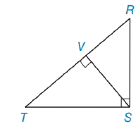Chapter 5.4, Problem 4EElementary Geometry For College St...

7th Edition
Alexander + 2 others
ISBN: 9781337614085

Solutions

Chapter
SectionElementary Geometry For College St...

7th Edition
Alexander + 2 others
ISBN: 9781337614085
Textbook Problem

Use theorem 5.4.3 to form a proportion in which TS is a geometric mean.[Hint: Δ T V S ∼ Δ T S R ]Exercises 1-6

To determine

To Find:

Proportion in which TS is the geometric mean.

Explanation

Given:

From the figure,

ΔRST is a right triangle with right angle RST, and SV¯ is the altitude to hypotenuse TR¯.

From the theorem,

The length of the altitude to the hypotenuse of a right triangle is the geometric mean of the lengths of the segments of the hypotenuse.

Since ΔRVSΔRST

Corresponding sides of similar triangles are proportional (CSSTP)

So, we have

VTTS=TSRT

Recall the Means-Extremes Property

In a proportion, the product of the means equals the product of the extremes

Still sussing out bartleby?

Check out a sample textbook solution.

See a sample solution

The Solution to Your Study Problems

Bartleby provides explanations to thousands of textbook problems written by our experts, many with advanced degrees!

Get Started

In Exercises 39-54, simplify the expression. (Assume that x, y, r, s, and t are positive.) 43. x3/4x1/4

Applied Calculus for the Managerial, Life, and Social Sciences: A Brief Approach

Consider the data 10, 12, 12, 8, 30, 12, 8, 10, 5, 23. 2. Find the median.

Mathematical Applications for the Management, Life, and Social Sciences

Rewritten as an iterated integral in polar coordinates,

Study Guide for Stewart's Multivariable Calculus, 8th

Graph each function. fx=x-13-3

College Algebra (MindTap Course List)

Define a construct and explain the role that constructs play in theories.

Research Methods for the Behavioral Sciences (MindTap Course List)